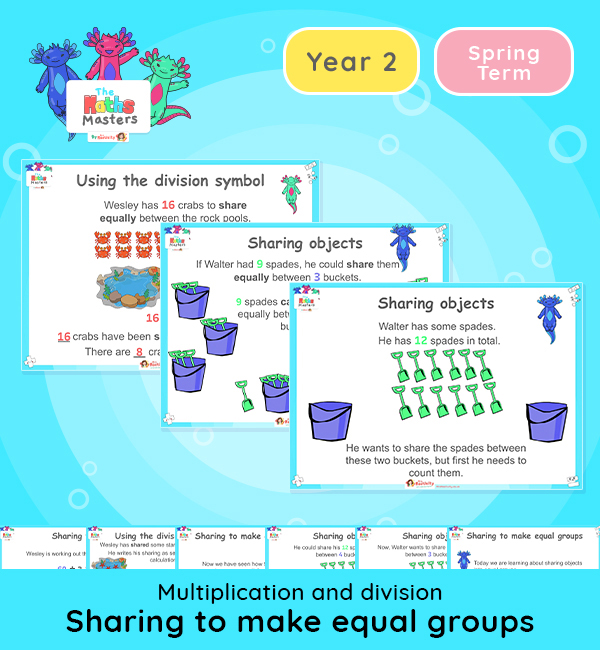# Year 2 | Sharing to Make Equal Groups Lesson Presentation## White Rose Year 2 multiplication and division lesson plans

Aligned with the White Rose Maths scheme of work, this Year 2 | Sharing to Make Equal Groups Lesson Presentation is fully editable, and is designed for the Year 2 maths curriculum covering the following maths objectives for the autumn term:

Topic: Multiplication and division

This lesson practises sharing numbers of objects to make equal groups. The first half recaps some of the learning from Year 1 about sharing objects into equal groups. The second half moves onto the Year 2 objective and introduces the use of the division symbol.

White Rose Maths Small Steps: Make equal groups – sharing.

NC Links: Recall and use multiplication and division facts for the 2, 5, and 10 times tables. *Calculate mathematical statements for multiplication and division within the multiplication tables and write them using the multiplication, division and equals signs. *Solve problems involving multiplication and division using materials, arrays, repeated addition, mental methods and multiplication and division facts including problems in context. *Count in steps of 2, 3, 5, and 10.

TAF Statements: Working At  – Recall and use multiplication and division facts for 2, 5, and 10 and use them to solve simple problems.

Greater Depth – Recall and use multiplication and division facts for 2, 5, and 10 and make deductions outside known multiplication facts. *Use reasoning about number and relationships to solve more complex problems and explain their thinking.

Ready-to-progress criteria:  2MD-2 Relate grouping problems where the number of groups is unknown to multiplication equation with a missing factor and two division equations (quotitive division)

Year 1 Conceptual prerequisites: Count in multiples of 2, 5, and 10 to find how many groups of 2, 5 or 10 there are in a particular quantity, set in everyday contexts.

## Recently Viewed